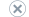# Specify a Column to Use as Regressor in a Model

Applies to:SQL Server 2019 and earlier Analysis ServicesAzure Analysis ServicesPower BI Premium

Important

Data mining was deprecated in SQL Server 2017 Analysis Services and now discontinued in SQL Server 2022 Analysis Services. Documentation is not updated for deprecated and discontinued features. To learn more, see Analysis Services backward compatibility.

A linear regression model represents the value of the predictable attribute as the result of a formula that combines the inputs in such a way that the data is fitted as a closely as possible to an estimated regression line. The algorithm accepts only numeric values as inputs, and automatically detects the inputs that provide the best fit.

However, you can specify that a column be included as a regressor by adding the FORCE_REGRESSOR parameter to the model and specifying the regressors to use. You might want to do this in cases where the attribute has meaning even if the effect is too small to be detected by the model, or when you want to ensure that the attribute is included in the formula.

The following procedure describes how to create a simple linear regression model, using the same sample data that is used for the neural networks tutorial. The model is not necessarily robust, but demonstrates how to use the Data Mining Designer to customize a linear regression model.

### How to create a simple linear regression model

1. In SQL Server Data Tools, in Solution Explorer, expand Mining Structures.

2. Double-click Call Center.dmm to open it in the designer.

3. From the Mining Model menu, select New Mining Model.

4. For the algorithm, select Microsoft Linear Regression. For the name, type Call Center Regression.

5. In the Mining Models tab, change the column usage as follows. All columns not in the following list should be set to Ignore, if they are not already.

FactCallCenterIDKey

Total OperatorsInput

AverageTimePerIssueInput

6. From the Mining Model menu, select Set Model Parameters.

7. For the parameter, FORCE_REGRESSOR, in the Value column, type the column names enclosed in brackets and separated by a comma, as follows:

``````[Average Time Per Issue],[Total Operators]
``````

Note

The algorithm will automatically detect which columns are the best regressors. You only need to force regressors when you want to ensure that a column is included in the final formula.

8. From the Mining Model menu, select Process Model.

In the viewer, the model is represented a single node containing the regression formula. You can view the formula in the Mining Legend, or you can extract the coefficients for the formula by using queries.